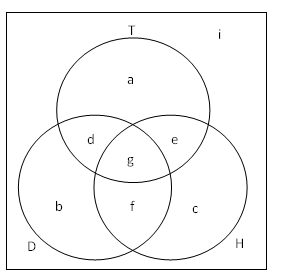# Venn Diagrams and Set Notation

A Venn Diagram is a way to visualize set relations between a finite number of sets. Below is a Venn Diagram for three sets $T, D,$ and $H$.Venn Diagram Sets

### We introduce some notation from Set Theory:

1. $|T|$ is the number of elements in set $T$.

2. Intersection of two sets, denoted $\cap$, refers to the elements that are in both sets. In the example, $T \cap D = \{ d, g\}$.

3. Union of two sets, denoted $\cup$, refers to the elements that are in at least one of the two sets. In the example, $T \cup H = \{a, c, d, e, f, g\}$.

4. Complement (Absolute), denoted $^c$, refers to the elements that are not in the set. In the example, $D^c = \{ a, c, e, i\}$.

5. Complement (Relative), denoted $\backslash$, refers to the elements in the first set, but are not in the second set. In the example, $H\backslash T = \{ c, f \}$.

6. Symmetric Difference, denoted $\triangle$, refers to the elements that are in at least one of the two sets, but are not in both sets. In the example, $D \triangle H = \{b, c, d, e\}$.Note by Arron Kau
7 years, 2 months ago

This discussion board is a place to discuss our Daily Challenges and the math and science related to those challenges. Explanations are more than just a solution — they should explain the steps and thinking strategies that you used to obtain the solution. Comments should further the discussion of math and science.

When posting on Brilliant:

• Use the emojis to react to an explanation, whether you're congratulating a job well done , or just really confused .
• Ask specific questions about the challenge or the steps in somebody's explanation. Well-posed questions can add a lot to the discussion, but posting "I don't understand!" doesn't help anyone.
• Try to contribute something new to the discussion, whether it is an extension, generalization or other idea related to the challenge.
• Stay on topic — we're all here to learn more about math and science, not to hear about your favorite get-rich-quick scheme or current world events.

MarkdownAppears as
*italics* or _italics_ italics
**bold** or __bold__ bold
- bulleted- list
• bulleted
• list
1. numbered2. list
1. numbered
2. list
Note: you must add a full line of space before and after lists for them to show up correctly
paragraph 1paragraph 2

paragraph 1

paragraph 2

[example link](https://brilliant.org)example link
> This is a quote
This is a quote
    # I indented these lines
# 4 spaces, and now they show
# up as a code block.

print "hello world"
# I indented these lines
# 4 spaces, and now they show
# up as a code block.

print "hello world"
MathAppears as
Remember to wrap math in $$ ... $$ or $ ... $ to ensure proper formatting.
2 \times 3 $2 \times 3$
2^{34} $2^{34}$
a_{i-1} $a_{i-1}$
\frac{2}{3} $\frac{2}{3}$
\sqrt{2} $\sqrt{2}$
\sum_{i=1}^3 $\sum_{i=1}^3$
\sin \theta $\sin \theta$
\boxed{123} $\boxed{123}$

## Comments

Sort by:

Top Newest

H\T can also be written H - T.

- 6 years, 10 months ago

Log in to reply

Ya ......dear right

- 6 years, 4 months ago

Log in to reply

Complement of H = H'

- 5 years ago

Log in to reply

As a non-mathematician I found some of the thinking in these examples quite puzzling and hard to follow!

- 3 years, 10 months ago

Log in to reply

The simplest things are the hardest :]

- 1 year, 4 months ago

Log in to reply

how do you solve when there is an unknown is a given set?

- 6 years, 11 months ago

Log in to reply

yeah,, idont find this post useful in solving,, please how do you actually solve???

- 6 years, 10 months ago

Log in to reply

You need to turn the rules into English and use logic. Like in the question that you probably came from, it says that: 0 is in the set. If $p$ is in the set, and $q$ is in the set, and $p \neq q$, then $p+q$ is in the set. Now, if 10 is in the set, then -10 being in the set wouldn't break any rules, becuase $-10 \neq 10$ and $-10+10=0$ which is also in the set.

Set notation isn't useful in problem solving, but it helps show the question in a short way. Imagine if we didn't have any math symbols. Then $3x-9= 0, x=3$ would be: "Take a number. If you take away nine from 3 times that number the answer is zero. The number is 3." Which is easier to understand?

- 2 years, 11 months ago

Log in to reply

Enlightening

- 3 years, 6 months ago

Log in to reply

this is kinda like logic gates

- 3 years, 3 months ago

Log in to reply

×

Problem Loading...

Note Loading...

Set Loading...